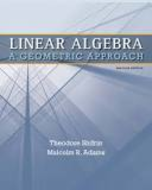Linear Algebra: A Geometric Approach - 2 Edition - Chapter 3.6 - Problem 6
Register Now

Join StudySoup

Get Full Access to Linear Algebra: A Geometric Approach - 2 Edition - Chapter 3.6 - Problem 69781429215213

# Decide whether each of the following is a subspace of C0(R). If so, provide a basis and

Linear Algebra: A Geometric Approach | 2nd Edition

Problem 6

Decide whether each of the following is a subspace of C0(R). If so, provide a basis and determine its dimension. If not, give a reason. a. {f : f (1) = 2} b. {f P2 : + 1 0 f (t)dt = 0} c. {f C1(R) : f _ (t) + 2f (t) = 0 for all t} d. {f P4 : f (t) tf _ (t) = 0 for all t} e. {f P4 : f (t) tf _ (t) = 1 for all t} f. {f C2(R) : f __ (t) + f (t) = 0 for all t} g. {f C2(R) : f __ (t) f _ (t) 6f (t) = 0 for all t} h. {f C1(R) : f (t) = + t 0 f (s)ds for all t}

Accepted Solution
Step-by-Step Solution:

Problem 6

Decide whether each of the following is a subspace of. If so, provide a basis and determine its dimension. If not, give a reason

a.b.c.d.e.f.g.h.Step by Step Solution

Step 1 of 8

(a)

Let us denote the given set byi.e.It is known that a non-empty subsetof a vector spaceis a subspace ofif:

***From the given definition of, it is clear that the zero functiondoesn’t belong toas it doesn’t satisfy the. Therefore,is not a subspace.

###### Chapter 3.6, Problem 6 is Solved

Step 2 of 8

Step 3 of 8

Unlock Textbook Solution